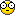(Tutorial) Changing texture on teleport for 4GW with MCS(Tutorial) Changing texture on teleport for 4GW with MCS

It it tutorial so as changing floor/ceiling color on teleport,but with texture. If you'd like use this tutorial 1st you must enable textured floor/ceiling function on 4GW and teleport function:

Textured floor/ceiling for 4GW:

http://wolf3dfanboys.forumotion.com/t142-tutorial-darkone-s-textured-floor-ceiling-for-4gw

Teleport:http://www.areyep.com/Codingtips/teleporter1.html

WL_DEF.H (gamestate):

Code:
`boolean                 warped;`

WL_MAIN.CPP (NewGame) and WL_GAME.CPP (Died):

Code:
`gamestate.warped = 0;`

WL_AGENT.CPP (WarpPlayer):

Code:
`gamestate.warped ^= 1;`

WL_DRAW.CPP (vgaCeiling under):

Code:
`unsigned vgaCeilingWarped[]={#ifndef SPEAR // 1      2      3      4      5      6      7      8      9      B      D// alte erste Zeile 0x9e9e,0x1d1d,0xdddd,0x1d1d,0x1e1e,0xd7d7,0xdede,0x9e9e,0x1d1d,0x1c1c,0x6565,  USE_TEX(15),USE_TEX(12),USE_TEX(12),USE_TEX(12),USE_TEX(12),USE_TEX(17),USE_TEX(12),USE_TEX(17),USE_TEX(17),USE_TEX(19), USE_TEX(24),USE_TEX(24),USE_TEX(24),USE_TEX(24),USE_TEX(24),USE_TEX(19),USE_TEX(12),USE_TEX(12),USE_TEX(15),USE_TEX(8), USE_TEX(12),USE_TEX(12),USE_TEX(12),USE_TEX(12),USE_TEX(12),USE_TEX(17),USE_TEX(12),USE_TEX(12),USE_TEX(12),USE_TEX(8), USE_TEX(17),USE_TEX(8),USE_TEX(17),USE_TEX(12),USE_TEX(29),USE_TEX(8),USE_TEX(17),USE_TEX(24),USE_TEX(35),USE_TEX(29), USE_TEX(35),USE_TEX(35),USE_TEX(35),USE_TEX(35),USE_TEX(12),USE_TEX(29),USE_TEX(35),USE_TEX(35),USE_TEX(35),USE_TEX(17), USE_TEX(17),USE_TEX(35),USE_TEX(35),USE_TEX(35),USE_TEX(35),USE_TEX(29),USE_TEX(29),USE_TEX(29),USE_TEX(29),USE_TEX(17)#else USE_TEX(54),USE_TEX(24),USE_TEX(12),USE_TEX(63),USE_TEX(50),USE_TEX(17),USE_TEX(8),USE_TEX(8),USE_TEX(8),USE_TEX(8), USE_TEX(35),USE_TEX(44),USE_TEX(29),USE_TEX(17),USE_TEX(12),USE_TEX(50),USE_TEX(50),USE_TEX(42),USE_TEX(63),USE_TEX(54),USE_TEX(56)#endif};`

(ThreeDRefresh):

Code:
` if(gamestate.warped)      ceiling=vgaCeilingWarped[gamestate.episode*10+mapon];    else        ceiling=vgaCeiling[gamestate.episode*10+mapon];`

Note:If you add teleport then you must change some code for 4GW because you'll get errors.

WL_AGENT.CPP (WarpPlayer):

Code:
`void WarpPlayer (int facedir){objtype  *check;int  x,y;buttonstate[bt_attack] = false;buttonheld[bt_attack] = false;gamestate.attackframe = 0;gamestate.weaponframe = 0;ThreeDRefresh();switch (facedir)  {   case EAST:   check = actorat[warpex][warpey];   if (check > objlist && (check->flags & FL_SHOOTABLE))    KillActor(check);   SpawnPlayer(warpex,warpey,facedir);   break;  case WEST:   check = actorat[warpwx][warpwy];   if (check > objlist && (check->flags & FL_SHOOTABLE))    KillActor(check);   SpawnPlayer(warpwx,warpwy,facedir);   break;  } SD_PlaySound (BOSSACTIVESND); if(DigiMode != sds_Off)  Delay (35); //adjust warp duration here else  VW_WaitVBL(1*50);buttonstate[bt_attack] = false;buttonheld[bt_attack] = false;gamestate.attackframe = 0;gamestate.weaponframe = 0;ThreeDRefresh();}`

USE_TEX(15) 1:An example to texture changing on teleport.

Thanks again to Chris the help. Credit to MCS it is function.Re: (Tutorial) Changing texture on teleport for 4GW with MCS

This is a very big list of codes!!!!Last edited by Ferir987 on Thu Jun 15, 2017 6:13 pm; edited 1 time in total (Reason for editing : editing problems,)

Vendég
GuestRe: (Tutorial) Changing texture on teleport for 4GW with MCS

Ferir987 wrote:This is a very big list of codes!!!!Oh yeah. Some code is long.Re: (Tutorial) Changing texture on teleport for 4GW with MCS

And you can remember all of it?Vendég
GuestRe: (Tutorial) Changing texture on teleport for 4GW with MCS

Ferir987 wrote:And you can remember all of it?For some code tutorial.Re: (Tutorial) Changing texture on teleport for 4GW with MCS